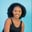Related Tags

python programming

# How to use the np.remainder() function for 2D array in PythonMaria Elijah

## Overview

The Python library NumPy has a method called remainder(). It is used to get the remainder of dividing two input arrays.

### The numpy.remainder()method

The numpy.remainder() method returns the remainder by dividing two input arrays. This is done element-wise (element by element).

Note: A two-dimensional (2D) array can be created using a list of lists in Python.

### Syntax

numpy.remainder(x1, x2, dtype=None, out=None)


### Parameters

• x1: An array_like that represents a dividend array.
• x2: An array_like that represents a divisor array.
• dtype: This is an optional parameter. It represents the return type of the array.
• out: This is an optional parameter. It represents the alternative output array in which the result is to be placed.

Note: If x1 and x2 have distinct shapes, they must be broadcastable to a common shape for output representation.

### Return value

The numpy.remainder() method returns the remainder of dividing two input arrays x1/x2.

Note: In Python, the remainder() function is equivalent to the modulus operator x1 % x2.

### Code example

The following code shows how to use the numpy.remainder() method for two-dimensional (2D) arrays:

# import numpy
import numpy as np
# create a two 2D arrays
x1 = np.array([[2,6,5],[3,4,8]])
x2 = np.array([[1,7,2],[10,9,4]])

# divide the 2D arrays
# and store the remainder in result
result = np.remainder(x1, x2)

print(result)
Calculating the remainder

### Code explanation

• Line 2: We import the numpy library.
• Lines 4 and 5: We create two 2D arrays called x1, and x2.
• Line 9: We use the np.remainder() method, which returns an array containing the remainder of dividing array x1 by array x2. The result is stored in a new variable called result.
• Line 11: We display the result.

RELATED TAGS

python programming

CONTRIBUTORMaria Elijah
RELATED COURSES

View all Courses

Keep Exploring

Learn in-demand tech skills in half the time